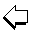Relations between optimized IOL constantsULIB User Group for Laser Interference BiometryConversion of IOL constants into one another

 Which constant is to be converted ? A-const (SRK II) A-const (SRK/T) pACD (HofferQ) sf (Holladay) a0 (Haigis) Value of constant to be converted : As decimal separator, please use '.' instead of ',' ! Click here to start calculation !

Relations between manufacturers' and optimized IOL constants
(W.Haigis)
IOL constants like A-constants or ACD-constants are given by lens manufacturers and are meant for an average measurement set-up. A given IOL is represented by different constants in the different IOL formulas. In the literature (e.g. ,,), there are simple transformation formulas ('standard relations') to calculate one IOL constant from another. This is the standard situation and the respective constants may be considered as default constants.
For a given surgeon's measurement setup it is highly probable that the published IOL constants are less than optimum. Therefore, it is commonly agreed that the published constants must be individualized, personalized, or optimized to make allowance for different measurement settings (e.g. different combinations of A-scans and keratometers).
Once constants are optimized, the classical (standard) relations given for (default) IOL constants break down and are replaced by new ones .
If optimized constants are not yet available, manufacturers' constants have to be used. Likewise, to derive the IOL constants for different formulas, the classical (standard) relations between constants have to be applied. IOL results with different formulas will in general differ from each other (most pronounced for the Haigis formula); usually, in cases where other formulas show a hyperopic tendency, the Haigis formula will produce more myopic results.
It is definitely preferable to use optimized IOL constants, which will produce a mean zero prediction error for all formulas. Formula differences will now show up for short and long eyes (cf e.g. ). If an optimized constant exists for one formula, the respective constants for the other formulas can now easily be calculated by means of the 'Relations between optimized constants.'

References:
: RETZLAFF J, SANDERS DR, KRAFF MC (1990): Lens Implant Power Calculation - A manual for ophthalmologists & biometrists, 3rd edition, Slack Inc, Thorofare NJ, USA
: HAIGIS W, LEGE B, MILLER N, SCHNEIDER B: Comparison of immersion ultrasound biometry and partial coherence interferometry for IOL calculation according to Haigis, Graefes Arch Clin Exp Ophthalmol (2000) 238:765-773
: HOLLADAY, JT: International intraocular lens implant registry 2003. J Cataract Refract Surg (2003) 29:176-197
: HAIGIS W: Relations between optimized IOL constants. Symposium on Cataract, IOL and Refractive Surgery of the American Society of Cataract and Refractive Surgery (ASCRS), Philadelphia, PA, USA, June 1-5, 2002, Abstracts, p.112, 2002
: http://www.doctor-hill.com/formulas.htmULIB Home
Last Revision: Jun 28, 2016 WH